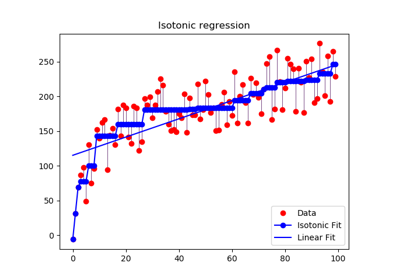# sklearn.isotonic.IsotonicRegression¶

class sklearn.isotonic.IsotonicRegression(y_min=None, y_max=None, increasing=True, out_of_bounds='nan')[source]

Isotonic regression model.

The isotonic regression optimization problem is defined by:

min sum w_i (y[i] - y_[i]) ** 2

subject to y_[i] <= y_[j] whenever X[i] <= X[j]
and min(y_) = y_min, max(y_) = y_max

where:
• y[i] are inputs (real numbers)

• y_[i] are fitted

• X specifies the order. If X is non-decreasing then y_ is non-decreasing.

• w[i] are optional strictly positive weights (default to 1.0)

Read more in the User Guide.

New in version 0.13.

Parameters
y_minoptional, default: None

If not None, set the lowest value of the fit to y_min.

y_maxoptional, default: None

If not None, set the highest value of the fit to y_max.

increasingboolean or string, optional, default: True

If boolean, whether or not to fit the isotonic regression with y increasing or decreasing.

The string value “auto” determines whether y should increase or decrease based on the Spearman correlation estimate’s sign.

out_of_boundsstring, optional, default: “nan”

The out_of_bounds parameter handles how x-values outside of the training domain are handled. When set to “nan”, predicted y-values will be NaN. When set to “clip”, predicted y-values will be set to the value corresponding to the nearest train interval endpoint. When set to “raise”, allow interp1d to throw ValueError.

Attributes
X_min_float

Minimum value of input array X_ for left bound.

X_max_float

Maximum value of input array X_ for right bound.

f_function

The stepwise interpolating function that covers the input domain X.

Notes

Ties are broken using the secondary method from Leeuw, 1977.

References

Isotonic Median Regression: A Linear Programming Approach Nilotpal Chakravarti Mathematics of Operations Research Vol. 14, No. 2 (May, 1989), pp. 303-308

Isotone Optimization in R : Pool-Adjacent-Violators Algorithm (PAVA) and Active Set Methods Leeuw, Hornik, Mair Journal of Statistical Software 2009

Correctness of Kruskal’s algorithms for monotone regression with ties Leeuw, Psychometrica, 1977

Examples

>>> from sklearn.datasets import make_regression
>>> from sklearn.isotonic import IsotonicRegression
>>> X, y = make_regression(n_samples=10, n_features=1, random_state=41)
>>> iso_reg = IsotonicRegression().fit(X.flatten(), y)
>>> iso_reg.predict([.1, .2])
array([1.8628..., 3.7256...])


Methods

 fit(self, X, y[, sample_weight]) Fit the model using X, y as training data. fit_transform(self, X[, y]) Fit to data, then transform it. get_params(self[, deep]) Get parameters for this estimator. predict(self, T) Predict new data by linear interpolation. score(self, X, y[, sample_weight]) Return the coefficient of determination R^2 of the prediction. set_params(self, \*\*params) Set the parameters of this estimator. transform(self, T) Transform new data by linear interpolation
__init__(self, y_min=None, y_max=None, increasing=True, out_of_bounds='nan')[source]

Initialize self. See help(type(self)) for accurate signature.

fit(self, X, y, sample_weight=None)[source]

Fit the model using X, y as training data.

Parameters
Xarray-like of shape (n_samples,)

Training data.

yarray-like of shape (n_samples,)

Training target.

sample_weightarray-like of shape (n_samples,), default=None

Weights. If set to None, all weights will be set to 1 (equal weights).

Returns
selfobject

Returns an instance of self.

Notes

X is stored for future use, as transform needs X to interpolate new input data.

fit_transform(self, X, y=None, **fit_params)[source]

Fit to data, then transform it.

Fits transformer to X and y with optional parameters fit_params and returns a transformed version of X.

Parameters
Xnumpy array of shape [n_samples, n_features]

Training set.

ynumpy array of shape [n_samples]

Target values.

**fit_paramsdict

Returns
X_newnumpy array of shape [n_samples, n_features_new]

Transformed array.

get_params(self, deep=True)[source]

Get parameters for this estimator.

Parameters
deepbool, default=True

If True, will return the parameters for this estimator and contained subobjects that are estimators.

Returns
paramsmapping of string to any

Parameter names mapped to their values.

predict(self, T)[source]

Predict new data by linear interpolation.

Parameters
Tarray-like of shape (n_samples,)

Data to transform.

Returns
T_array, shape=(n_samples,)

Transformed data.

score(self, X, y, sample_weight=None)[source]

Return the coefficient of determination R^2 of the prediction.

The coefficient R^2 is defined as (1 - u/v), where u is the residual sum of squares ((y_true - y_pred) ** 2).sum() and v is the total sum of squares ((y_true - y_true.mean()) ** 2).sum(). The best possible score is 1.0 and it can be negative (because the model can be arbitrarily worse). A constant model that always predicts the expected value of y, disregarding the input features, would get a R^2 score of 0.0.

Parameters
Xarray-like of shape (n_samples, n_features)

Test samples. For some estimators this may be a precomputed kernel matrix or a list of generic objects instead, shape = (n_samples, n_samples_fitted), where n_samples_fitted is the number of samples used in the fitting for the estimator.

yarray-like of shape (n_samples,) or (n_samples, n_outputs)

True values for X.

sample_weightarray-like of shape (n_samples,), default=None

Sample weights.

Returns
scorefloat

R^2 of self.predict(X) wrt. y.

Notes

The R2 score used when calling score on a regressor will use multioutput='uniform_average' from version 0.23 to keep consistent with r2_score. This will influence the score method of all the multioutput regressors (except for MultiOutputRegressor). To specify the default value manually and avoid the warning, please either call r2_score directly or make a custom scorer with make_scorer (the built-in scorer 'r2' uses multioutput='uniform_average').

set_params(self, **params)[source]

Set the parameters of this estimator.

The method works on simple estimators as well as on nested objects (such as pipelines). The latter have parameters of the form <component>__<parameter> so that it’s possible to update each component of a nested object.

Parameters
**paramsdict

Estimator parameters.

Returns
selfobject

Estimator instance.

transform(self, T)[source]

Transform new data by linear interpolation

Parameters
Tarray-like of shape (n_samples,)

Data to transform.

Returns
T_array, shape=(n_samples,)

The transformed data

## Examples using sklearn.isotonic.IsotonicRegression¶